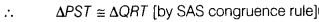# Given figure, PQRS is a square and SRT is an equilateral triangle. Prove that PT = QT

In the given figure, PQRS is a square and SRT is an equilateral triangle. Prove that PT = QT.

Since, POPS is a square and ∆SRT is an equilateral triangle.
∴ ∠PSR = 90° and ∠TSR = 60°
=> ∠PSR + ∠TSR = 90°+ 60°
=> ∠PST = 150°
Similarly, we have
∠QRT = 150°
Thus, in A PST and ∆ QRT, we have
PS = QR [sides of a square]
∠PST = ∠QRT = 150°
and ST = RT [sides of an equilateral triangle]=> PT =QT